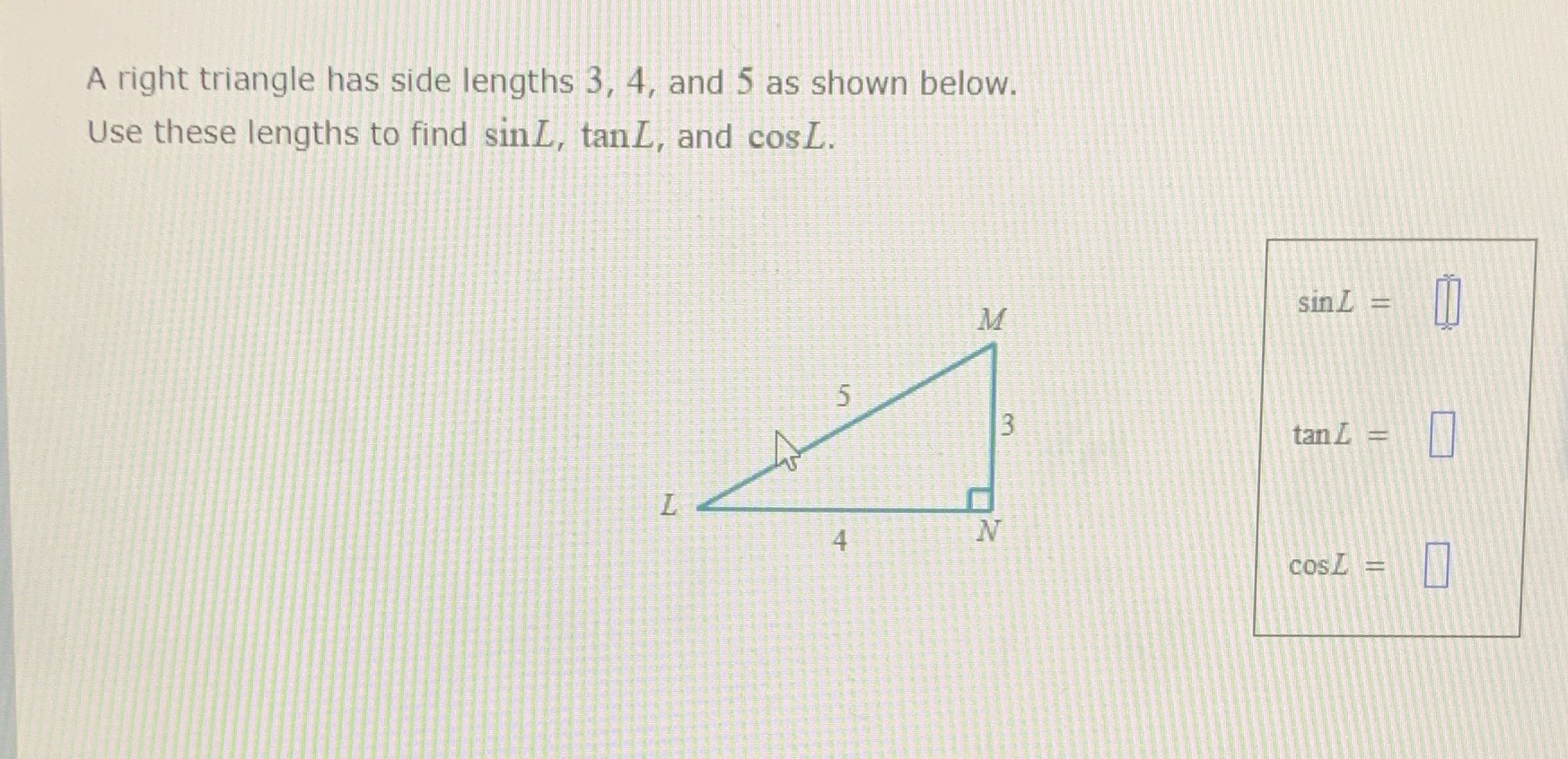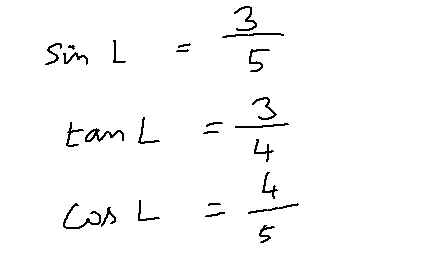### Still have math questions?A right triangle has side lengths $$3,4 ,$$ and $$5$$ as shown below. Use these lengths to find $$\sin L , \tan L ,$$ and $$\cos L$$ .